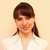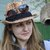# "Excel" category, page 13## How to insert dates in Excel - add today's date, auto fill a column with dates

This tutorial demonstrates various ways of entering dates in Excel. See how to insert today's date and current time as static or dynamic values, how to auto populate a column or row with weekdays, and how to auto fill random dates in Excel. Continue reading## How to change date format in Excel and create custom formatting

The first part of our tutorial focuses of formatting dates in Excel and explains how to set the default date and time formats, how to change date format in Excel, how to create custom date formatting, and convert your dates to another locale. Continue reading## Excel array formula examples for beginners and advanced users

The tutorial focuses on Excel array formula examples and demonstrates how to use Excel functions that support calculations in arrays to count cells that meet several conditions, sum values in every Nth row, count any given character in a range, and more. Continue reading## Excel array formulas, functions and constants - examples and guidelines

In this tutorial, you will learn what an Excel array formula is, how to enter it correctly in your worksheets, and how to use array constants and array functions in Excel. Continue reading## How to count the number of characters in Excel cells

Initially, Excel was designed to work with numbers. Fortunately, the developers of this helpful application didn't forget about text. Below, you'll find a number of formulas for counting characters in Excel. Just look through the examples and see which one best suits your needs. Continue reading## INDEX function in Excel - 6 most efficient uses

In this tutorial, you will find a number of Excel INDEX formula examples that demonstrate the most efficient uses of INDEX in Excel. Continue reading## Using OFFSET function in Excel - formula examples

The tutorial explains the arcane twists of the OFFSET function and covers the most efficient ways to use OFFSET in Excel. Continue reading## How to use INDIRECT function in Excel - formula examples

This Excel INDIRECT tutorial explains the function's syntax, basic uses and provides a number of formula examples that demonstrate how to use INDIRECT in Excel. Continue reading## Excel templates: how to make and use

Learn how to make your own Excel templates and where to find the best collections of free templates for your Excel such as calendars, budgets, invoices, inventory, charts, and more. Continue reading## How to calculate compound interest in Excel: daily, monthly, yearly compounding

The tutorial explains the compound interest formula for Excel and provides examples of how to calculate the future value of the investment at annual, monthly or daily compounding interest rate. You will also find the detailed steps to create your own Excel compound interest calculator. Continue reading## How to calculate percentage in Excel - formula examples

In this tutorial, you will lean a quick way to calculate percentages in Excel, find the basic percentage formula and a few more formulas for calculating percentage increase, percent of total and more. Continue reading## How to show percentages in Excel

In this short tutorial, you will find many helpful details about Excel percent format and learn how to make Excel display percentages in the way you want. ﻿ Continue reading## Using logical functions in Excel: AND, OR, XOR and NOT

Last week we tapped into the insight of Excel logical operators that are used to compare data in different cells. Today, you will see how to extend the use of logical operators and construct more elaborate logical tests to perform more complex calculations and more powerful data analysis. Excel logical functions such as AND, OR, XOR and NOT will help you in doing this. Continue reading## Excel logical operators: equal to, not equal to, greater than, less than

Many tasks you perform in Excel involve comparing data in different cells. For this, Microsoft Excel provides six logical operators, which are also called comparison operators. This tutorial aims to help you understand the insight of Excel logical operators and write the most efficient formulas for your data analysis. Continue reading## Excel IF statement with multiple conditions

For powerful data analysis, you may often need to build an Excel IF statement with multiple conditions or use IF together with other functions. This tutorial will show you the most effective ways to do this. Continue reading## How to use IF function in Excel: examples for text, numbers, dates, blanks

IF is one of the most popular and useful functions in Excel. Generally, you use an IF statement to test a condition and to return one value if the condition is met, and another value if the condition is not met. Continue reading## How to use Pivot Tables in Excel - tutorial for beginners

In this Excel pivot table tutorial you will learn what a PivotTable is, find a number of pivot table examples that will help you to get started quickly and see how to create and use pivot tables in Excel 2016, 2013, 2010 and 2007. Continue reading## How to use Excel SUMIFS and SUMIF with multiple criteria

This tutorial explains the difference between the SUMIF and SUMIFS functions in terms of their syntax and usage, and provides a number of formula examples to sum values with multiple AND / OR criteria in Excel. Continue reading## How to use SUMIF function in Excel with formula examples

If you are faced with a task that requires conditional sum in Excel, the SUMIF function is what you need. It is a really great function that can help you make sense of an incomprehensible set of diverse data. Instead of adding up all numbers in a range, it lets you sum only those values that meet your criteria. Continue reading## 5 ways to sum a column in Excel

This tutorial shows how to sum a column in Excel 2010-2013. Try out 5 different ways to total columns: find the sum of the selected cells on the Status bar, use AutoSum in Excel to sum all or only filtered cells, employ the SUM function or convert your range to Table for easy calculations. Continue reading## Converting PDF files (text and image) to Excel

The article explains how to export different PDF files to Excel manually or using free online converters, and how to choose the conversion method best suited for a given file type. Continue reading## How to convert numbers to text using Excel TEXT function and other options

This tutorial shows how to convert number to text in Excel 2016 - 2010. See how to accomplish the task with the Excel TEXT function and use number to string to specify the formatting. Learn how to change number format to text with the Format Cells… and Text to Columns options. Continue reading## How to convert Excel files to PDF

This short tutorial describes 4 possible ways to convert Excel files to PDF – by using Excel's Save As feature, Adobe software, online Excel to PDF converters and desktop tools. Continue reading## Making a dependent (cascading) drop down list in Excel

The tutorial demonstrates how to create cascading drop down lists in Excel that display choices depending on the value selected in a previous dropdown. Continue reading## How to create drop down list in Excel from range, table, another workbook

The tutorial demonstrates 4 quick ways to create an Excel drop down list - based on a list of values, range of cells, named range and a dynamic dropdown. Continue reading## How to insert new columns in Excel

This post looks at how to add up new columns in Excel. Read on to learn shortcuts to insert one or more columns, including nonadjacent ones. Grab and share a special VBA macros to automate adding every other column. Continue reading## Excel VLOOKUP not working - solving #N/A and #VALUE errors

The tutorial explains how you can quickly cope with VLOOKUP not working problems in Excel, troubleshoot and fix common errors and overcome VLOOKUP's limitations. Continue reading## How to remove rows in Excel based on a cell value

This article lists several ways to delete rows in Excel based on a cell value. In this post you'll find hotkeys as well as Excel VBA. Delete rows automatically or use the standard Sort and Find options in combination with helpful shortcuts. Continue reading## 5 ways to do case-sensitive VLOOKUP in Excel

The tutorial explains how to make Excel VLOOKUP case-sensitive, demonstrates a few other formulas that can look up case in Excel and points out strengths and limitations of each function. Continue reading## INDEX & MATCH in Excel - better alternative to VLOOKUP

This tutorial demonstrates the key strengths of Excel's INDEX / MATCH function that make it superior to VLOOKUP. You will find a number of formula examples that will help you easily cope with many complex tasks when VLOOKUP fails. Continue reading